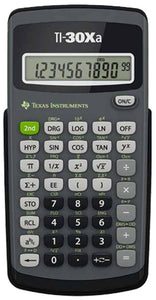TI-30XA Calculator

• \$14.99
Unit price per
Shipping calculated at checkout.

Exam acceptance

The TI-30Xa scientific calculator is approved for use on SAT, ACT, and AP exams.

Ideal for

• General math
• Pre-Algebra
• Algebra 1 and 2
• General science

Operations/Functionality:

• Fraction/decimal conversions
• Change improper fractions to mixed numbers
• Automatic simplification of fractions
• One constant
• Combinations and permutations
• Trigonometry
• Hyperbolics
• Logs and antilogs